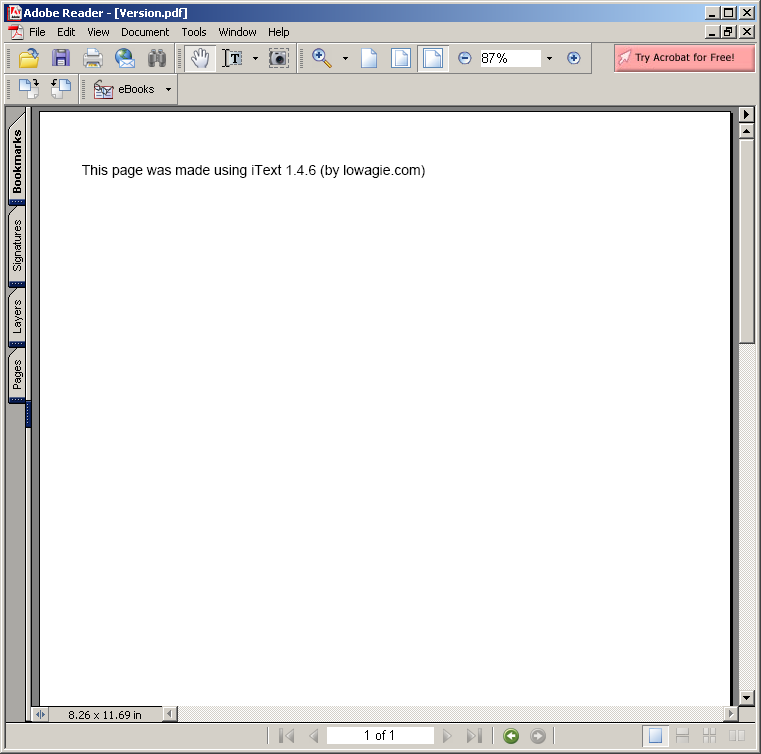# Find connections using a depth-first search : Algorithms « Collections Data Structure « Java

Find connections using a depth-first search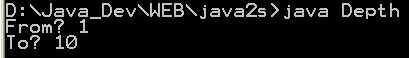```
/*
* Chapter 10 - AI-Based Problem Solving The Art of Java by Herbert Schildt and
* James Holmes McGraw-Hill/Osborne 2003
*
*/

//The entire depth-first search program follows:
//Find connections using a depth-first search.

import java.util.*;
import java.io.*;

//Flight information.
class FlightInfo {
String from;

String to;

int distance;

boolean skip; // used in backtracking

FlightInfo(String f, String t, int d) {
from = f;
to = t;
distance = d;
skip = false;
}
}

public class Depth {
final int MAX = 100; // maximum number of connections

// This array holds the flight information.
FlightInfo flights[] = new FlightInfo[MAX];

int numFlights = 0; // number of entries in flight array

Stack btStack = new Stack(); // backtrack stack

public static void main(String args[]) {
String to, from;
Depth ob = new Depth();

ob.setup();

try {
System.out.print("From? ");
System.out.print("To? ");

ob.isflight(from, to);

if (ob.btStack.size() != 0)
ob.route(to);
} catch (IOException exc) {
System.out.println("Error on input.");
}
}

//Initialize the flight database.
void setup() {
addFlight("New York", "Chicago", 900);
addFlight("New York", "Toronto", 500);
addFlight("New York", "Denver", 1800);
addFlight("Toronto", "Los Angeles", 2500);
addFlight("Houston", "Los Angeles", 1500);
addFlight("Denver", "Los Angeles", 1000);
}

//Put flights into the database.
void addFlight(String from, String to, int dist) {
if (numFlights < MAX) {
flights[numFlights] = new FlightInfo(from, to, dist);

numFlights++;
} else
System.out.println("Flight database full.\n");
}

// Show the route and total distance.
void route(String to) {
Stack rev = new Stack();
int dist = 0;
FlightInfo f;
int num = btStack.size();

// Reverse the stack to display route.
for (int i = 0; i < num; i++)
rev.push(btStack.pop());

for (int i = 0; i < num; i++) {
f = (FlightInfo) rev.pop();
System.out.print(f.from + " to ");
dist += f.distance;
}

System.out.println(to);
System.out.println("Distance is " + dist);
}

/*
* If there is a flight between from and to, return the distance of flight;
* otherwise, return 0.
*/
int match(String from, String to) {
for (int i = numFlights - 1; i > -1; i--) {
if (flights[i].from.equals(from) && flights[i].to.equals(to)
&& !flights[i].skip) {
flights[i].skip = true; // prevent reuse
return flights[i].distance;
}
}

}

// Given from, find any connection.
FlightInfo find(String from) {
for (int i = 0; i < numFlights; i++) {
if (flights[i].from.equals(from) && !flights[i].skip) {
FlightInfo f = new FlightInfo(flights[i].from, flights[i].to,
flights[i].distance);
flights[i].skip = true; // prevent reuse

return f;
}
}

return null;
}

// Determine if there is a route between from and to.
void isflight(String from, String to) {
int dist;
FlightInfo f;

// See if at destination.
dist = match(from, to);
if (dist != 0) {
btStack.push(new FlightInfo(from, to, dist));
return;
}

// Try another connection.
f = find(from);
if (f != null) {
btStack.push(new FlightInfo(from, to, f.distance));
isflight(f.to, to);
} else if (btStack.size() > 0) {
// Backtrack and try another connection.
f = (FlightInfo) btStack.pop();
isflight(f.from, f.to);
}
}
}

```

### Related examples in the same category

 1 Anagrams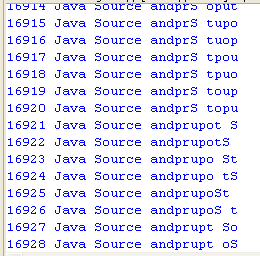2 Hanoi puzzle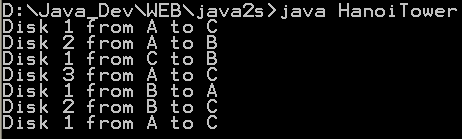3 Fibonacci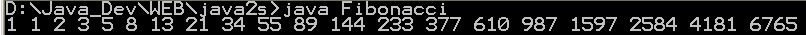4 Sieve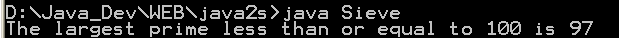5 Find connections using hill climbing. 6 Find optimal solution using least-cost 7 Find the lost keys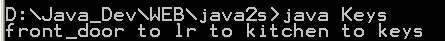8 Compute the area of a triangle using Heron's Formula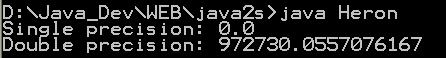9 Compute prime numbers 10 Print a table of fahrenheit and celsius temperatures 1 11 Print a table of fahrenheit and celsius temperatures 2 12 Print a table of Fahrenheit and Celsius temperatures 3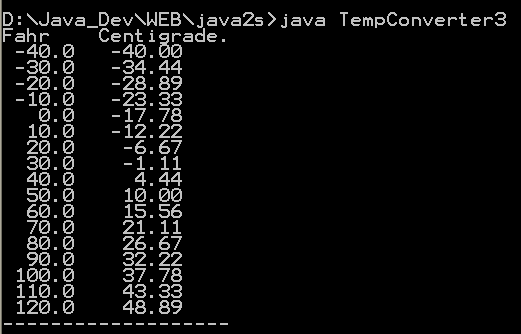13 Soundex - the Soundex Algorithm, as described by Knuth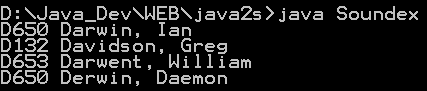14 A programmable Finite State Machine implementation. 15 An extendable Graph datastructure. 16 Utilities for flop (floating-point operation) counting. 17 LU Decomposition 18 Reverse Polish Notation 19 Permutator test 20 implements the LZF lossless data compression algorithm 21 Linear Interpolation 22 Utility class for generating the k-subsets of the numbers 0 to n 23 Version